• max函数
千次阅读
2021-05-01 01:37:33

这个Oracle教程解释了如何使用Oracle / PLSQL MAX函数。

Oracle / PLSQL MAX函数返回表达式的最大值。

MAX 函数对一组行进行操作，并返回一行输出。

您可以使用MAX具有任何有效表达式的函数。例如，您可以使用MAX()数字，字符串和数据时间。

空值将被忽略MAX。您可以使用DISTINCT关键字排除重复条目。

语法

Oracle / PLSQL MAX函数的语法是：

SELECT MAX( expression )

FROM tables

WHERE conditions;

expression 可以是数字字段或公式。

以下SQL返回所有员工的最高工资。

SELECT MAX(salary) AS "Highest Salary"

FROM employees;

以下示例使用MAX函数返回部门的名称和部门的最高工资。

SELECT department, MAX(salary) AS "Highest salary"

FROM employees

GROUP BY department;

实例

CREATE TABLE EMP (EMPNO NUMBER(4) NOT NULL,

ENAME VARCHAR2(10),

JOB VARCHAR2(9),

SAL NUMBER(7, 2),

DEPTNO NUMBER(2));

INSERT INTO EMP VALUES (1, 'SMITH', 'CLERK', 800, 20);

INSERT INTO EMP VALUES (2, 'ALLEN', 'SALESMAN', 1600, 30);

INSERT INTO EMP VALUES (3, 'WARD', 'SALESMAN', 1250, 30);

INSERT INTO EMP VALUES (4, 'JONES', 'MANAGER', 2975, 20);

INSERT INTO EMP VALUES (5, 'MARTIN','SALESMAN', 1250, 30);

INSERT INTO EMP VALUES (6, 'BLAKE', 'MANAGER', 2850, 30);

INSERT INTO EMP VALUES (7, 'CLARK', 'MANAGER', 2850, 10);

INSERT INTO EMP VALUES (8, 'SCOTT', 'ANALYST', 3000, 20);

INSERT INTO EMP VALUES (9, 'KING', 'PRESIDENT',3000, 10);

INSERT INTO EMP VALUES (10,'TURNER','SALESMAN', 1500, 30);

INSERT INTO EMP VALUES (11,'ADAMS', 'CLERK', 1500, 20);

SQL>

SQL> select max(empno) from emp;

MAX(EMPNO)

----------

11

SQL>

字符串值

当MAX()处理字符串时，字符串按字母顺序排列，最大字符串位于列表的底部。

SQL>

SQL> select max(ename) from emp;

MAX(ENAME)

----------

WARD

SQL>

使用MAX带group by子句的函数：

SQL> select max(sal) from emp group by deptno;

MAX(SAL)

----------

2850

3000

3000

SQL>

日期值

使用MAX与date值：

SQL> CREATE TABLE EMP (EMPNO NUMBER(4) NOT NULL,

2 ENAME VARCHAR2(10),

3 HIREDATE DATE);

Table created.

SQL>

SQL> INSERT INTO EMP VALUES (1, 'SMITH', TO_DATE('17-DEC-1980', 'DD-MON-YYYY'));

1 row created.

SQL> INSERT INTO EMP VALUES (2, 'ALLEN', TO_DATE('20-FEB-1981', 'DD-MON-YYYY'));

1 row created.

SQL> INSERT INTO EMP VALUES (3, 'WARD', TO_DATE('22-FEB-1981', 'DD-MON-YYYY'));

1 row created.

SQL> INSERT INTO EMP VALUES (4, 'JONES', TO_DATE('2-APR-1981', 'DD-MON-YYYY'));

1 row created.

SQL> INSERT INTO EMP VALUES (5, 'MARTIN',TO_DATE('28-SEP-1981', 'DD-MON-YYYY'));

1 row created.

SQL>

SQL> select max(HIREDATE) from emp;

MAX(HIRED

---------

28-SEP-81

SQL>

更多相关内容
• ## Matlab中max函数

千次阅读 2022-03-08 14:16:12
目录 语法 说明 示例 ...max函数式是求数组的最大元素。 语法 M = max(A) M = max(A,[],dim) M = max(A,[],nanflag) M = max(A,[],dim,nanflag) [M,I] = max(___) M = max(A,[],'all') M ...

目录

语法

说明

示例

向量中的最大元素

最大复数元素

每个矩阵列中的最大元素

每个矩阵行中的最大元素

存在 NaN 时的最大元素

最大元素索引

数组页面的最大值

返回线性索引

最大元素比较

max函数式是求数组的最大元素。

## 语法

M = max(A)
M = max(A,[],dim)
M = max(A,[],nanflag)
M = max(A,[],dim,nanflag)
[M,I] = max(___)
M = max(A,[],'all')
M = max(A,[],vecdim)
M = max(A,[],'all',nanflag)
M = max(A,[],vecdim,nanflag)
[M,I] = max(A,[],___,'linear')
C = max(A,B)
C = max(A,B,nanflag)

## 说明

M = max(A) 返回数组的最大元素。

如果 A 是向量，则 max(A) 返回 A 的最大值。

如果 A 为矩阵，则 max(A) 是包含每一列的最大值的行向量。

如果 A 是多维数组，则 max(A) 沿大小不等于 1 的第一个数组维度计算，并将这些元素视为向量。此维度的大小将变为 1，而所有其他维度的大小保持不变。如果 A 是第一个维度长度为零的空数组，则 max(A) 返回与 A 大小相同的空数组。

M = max(A,[],dim) 返回维度 dim 上的最大元素。例如，如果 A 为矩阵，则 max(A,[],2) 是包含每一行的最大值的列向量。

M = max(A,[],nanflag) 指定在计算中包括还是忽略 NaN 值。例如，max(A,[],'includenan') 包括 A 中的所有 NaN 值，而 max(A,[],'omitnan') 忽略这些值。

M = max(A,[],dim,nanflag) 还指定使用 nanflag 选项时的运算维度。

[M,I] = max(___) 在上述语法基础上，还返回 A 中最大值在运算维度上的对应索引。

M = max(A,[],'all') 查找 A 的所有元素的最大值。此语法适用于 MATLAB® R2018b 及更高版本。

M = max(A,[],vecdim) 计算向量 vecdim 所指定的维度上的最大值。例如，如果 A 是矩阵，则 max(A,[],[1 2]) 计算 A 中所有元素的最大值，因为矩阵的每个元素都包含在由维度 1 和 2 定义的数组切片中。

M = max(A,[],'all',nanflag) 计算在使用 nanflag 选项时 A 的所有元素的最大值。

M = max(A,[],vecdim,nanflag) 指定在使用 nanflag 选项时要运算的多个维度。

[M,I] = max(A,[],___,'linear') 返回 A 中最大值在 A 中的对应线性索引。

C = max(A,B) 返回从 A 或 B 中提取的最大元素的数组。

C = max(A,B,nanflag) 还指定如何处理 NaN 值。

## 示例

### 向量中的最大元素

创建一个向量并计算其最大元素。

A = [23 42 37 18 52];
M = max(A)
M = 52

### 最大复数元素

创建一个复数向量并计算其最大元素，即具有最大幅值的元素。

A = [-2+2i 4+i -1-3i];
max(A)
ans = 4.0000 + 1.0000i

### 每个矩阵列中的最大元素

创建一个矩阵并计算每列中的最大元素。

A = [2 8 4; 7 3 9]
A = 2×3

2     8     4
7     3     9

M = max(A)
M = 1×3

7     8     9

### 每个矩阵行中的最大元素

创建一个矩阵并计算每行中的最大元素。

A = [1.7 1.2 1.5; 1.3 1.6 1.99]
A = 2×3

1.7000    1.2000    1.5000
1.3000    1.6000    1.9900

M = max(A,[],2)
M = 2×1

1.7000
1.9900

### 存在 NaN 时的最大元素

创建一个向量并计算其最大值，不包括 NaN 值。

A = [1.77 -0.005 3.98 -2.95 NaN 0.34 NaN 0.19];
M = max(A,[],'omitnan')
M = 3.9800

由于 'omitnan' 是默认选项，因此 max(A) 也将生成此结果。使用 'includenan' 标志可返回 NaN。

M = max(A,[],'includenan')
M = NaN

### 最大元素索引

创建一个矩阵 A 并计算每列中的最大元素，以及这些元素在 A 中显示的行索引。

A = [1 9 -2; 8 4 -5]
A = 2×3

1     9    -2
8     4    -5

[M,I] = max(A)
M = 1×3

8     9    -2

I = 1×3

2     1     1

### 数组页面的最大值

创建一个三维数组并计算每页数据（行和列）的最大值。

A(:,:,1) = [2 4; -2 1];
A(:,:,2) = [9 13; -5 7];
A(:,:,3) = [4 4; 8 -3];
M1 = max(A,[],[1 2])
M1 =
M1(:,:,1) =

4

M1(:,:,2) =

13

M1(:,:,3) =

8

从 R2018b 开始，要计算一个数组的所有维度上的最大值，可以在向量维参数中指定每个维度，或使用 'all' 选项。

M2 = max(A,[],[1 2 3])
M2 = 13
Mall = max(A,[],'all')
Mall = 13

### 返回线性索引

创建一个矩阵 A，并返回矩阵 M 中每行的最大值。使用 'linear' 选项还可以返回线性索引 I，满足 M = A(I)。

A = [1 2 3; 4 5 6]
A = 2×3

1     2     3
4     5     6

[M,I] = max(A,[],2,'linear')
M = 2×1

3
6

I = 2×1

5
6

maxvals = A(I)
maxvals = 2×1

3
6

### 最大元素比较

创建一个矩阵并返回其各个元素与标量相比的最大值。

A = [1 7 3; 6 2 9]
A = 2×3

1     7     3
6     2     9

B = 5;
C = max(A,B)
C = 2×3

5     7     5
6     5     9

展开全文matlab
• 我们打开excel，随便写...就进入“插入函数”的设置页面，如下图所示，我们就是在这里找我们要使用的函数我们要使用的max函数是属于“统计”类别的，所以我们需要在“选择类别”后面的下拉框中选择“统计”选择“...

我们打开excel，随便写一些数据，作为我们要查找最大值的操作对象，如下图所示：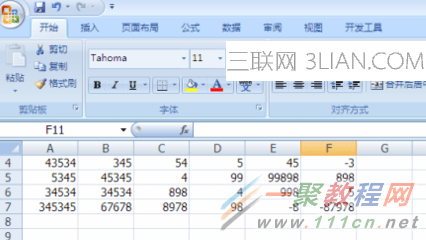我们做一点修改，输入“最大的数”，然后把光标停留在“最大的数”后面的一个单元格中，点击红色方框中的“fx”，可以进入函数设置页面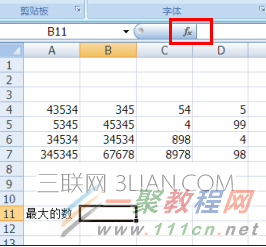点击“fx”后，就进入“插入函数”的设置页面，如下图所示，我们就是在这里找我们要使用的函数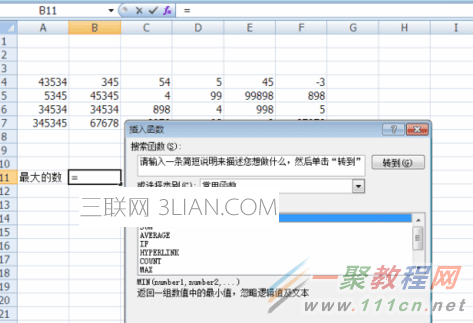我们要使用的max函数是属于“统计”类别的，所以我们需要在“选择类别”后面的下拉框中选择“统计”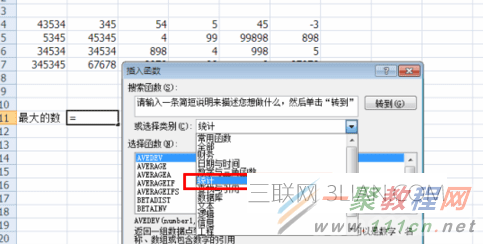选择“统计”之后，在“选择函数”下面的函数列表中列出的都是关于“统计”的函数，我们选择我们要使用的“MAX”，之后点右下方的“确定”。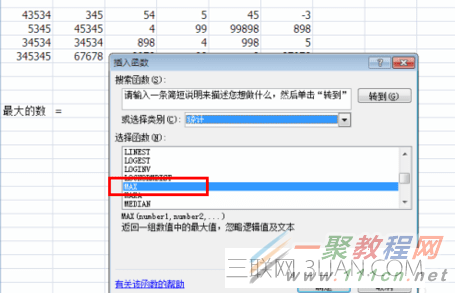完成第5步之后，就进入到“函数参数”的设置界面，我们删除Number1后面的内容，我们自己来选择我们的范围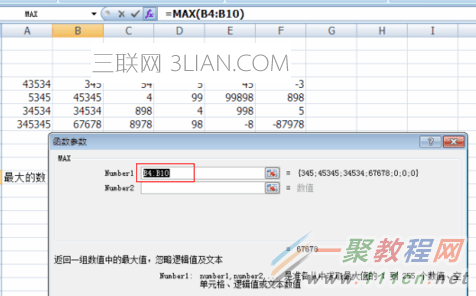假设我们要查找的范围是本表格中的所有数据，那么我们要设置的第一个参数应该是表格中第一个数据，我们用鼠标点击第一个数据的值，会发现第一个参数就自动帮我们填入到Number1后面的输入框中了，如下图所示：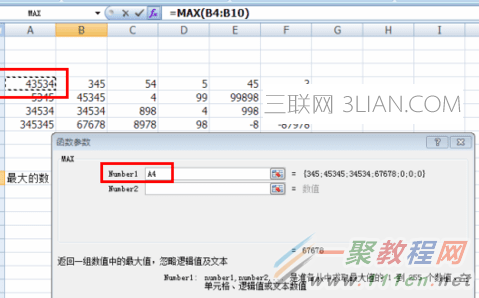之后我们在第一个参数后面手动输入冒号，冒号表示范围。如下图红色方框所示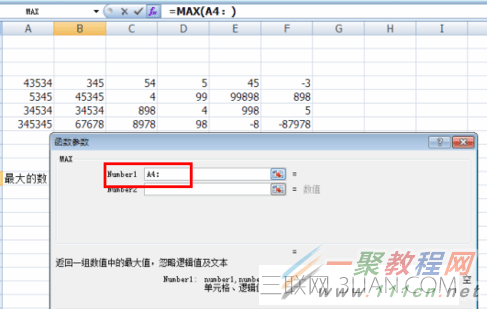现在我们要做的是设置最后一个参数了，我们只要用鼠标点击一下表格最后面的一个数据就可以了，excel就会自动帮我们填写这个参数的值。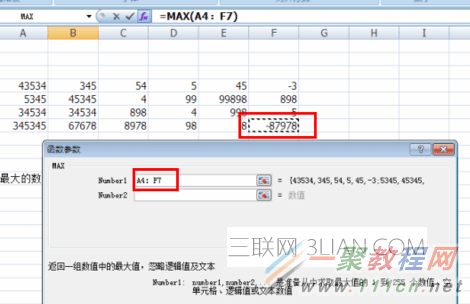所有的参数都设置好了，现在我们点“确定”按钮，就会关闭“函数参数”设置对话框，回到表格中，这是我们发现已经帮我们查找到最大的数值了。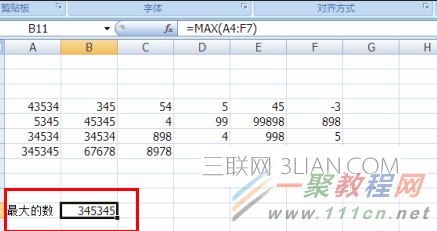展开全文• C++--问题35--min和max函数的用法 1.max函数 用于求数组中的最大元素。暂时用到的语法形式有以下3种： （1）C = max(A); （2）C = max(A, B); （3）C = max(A, [], dim); 描述： （1）C = max(A)返回数组不同...

# 1.max函数

用于求数组中的最大元素。暂时用到的语法形式有以下3种：

（1）C = max(A);

（2）C = max(A, B);

（3）C = max(A, [], dim);

描述：

（1）C = max(A)返回数组不同维度的最大元素。如果A是一个向量，max(A)返回向量A中的最大元素；如果A是一个矩阵，max(A)将矩阵A的每一列视为向量，最后返回一个行向量，这个行向量的每个元素是对应列向量的最大元素；如果A是一个多维数组，max(A)将第一个尺寸不为1的维度里的值视为向量，然后返回每个向量的最大值。

（2）C = max(A, B)返回一个数组，该数组的尺寸与A和B相同，该数组的元素取自A和B中的最大值。A和B的维度必须要匹配，或者它们都是标量。

（3）C = max(A, [], dim)根据指定标量dim返回矩阵A中的最大元素。比如dim=1，则返回行向量，其中每个元素都是对应列的最大元素；比如dim=2，则返回列向量，其中每个元素都是对应行的最大元素。

语法1举例1：在数组中返回最大的数字

A = [1, 2, 3, 4, 5]
A = 1 2 3 4 5
>>max(A)
ans = 5

语法1举例2：在5*5的矩阵中，每列中找到最大的数返回成一个数组

>>A = magic(5)
A =
17    24     1     8    15
23     5     7    14    16
4     6    13    20    22
10    12    19    21     3
11    18    25     2     9
>>max(A)
ans = 23 24 25 21 22
语法2举例1：两个二维数组（矩阵），维数相同，返回每个位置的最大值，返回一个二维数组

>>A = [1, 2, 3; 4, 5, 6; 7, 8, 9]
A =
1     2     3
4     5     6
7     8     9
>>B = [9, 8, 7; 6, 5, 4; 3, 2, 1]
B =
9     8     7
6     5     4
3     2     1
>>max(A, B)
ans =
9     8     7
6     5     6
7     8     9

语法2举例2：二维数组的每个数都和5比较，返回一个二维数组
>>A = magic(3)
A =
8     1     6
3     5     7
4     9     2
>>max(A, 5)
ans =
8     5     6
5     5     7
5     9     5
语法3举例：第三个参数的1代表以列为单位比较，返回没一列的最大值组成行的数组，
第三个参数的2代表以行为单位比较，返回没一行的最大值组成列的数组。
>>A = magic(3)
A =
8     1     6
3     5     7
4     9     2
>>max(A, [], 1)
ans = 8 9 7
>>max(A, [], 2)
ans =
8
7
9

# 2.min函数

用于求数组中的最小值。暂时用到的语法形式有3种：

（1）C = min(A);

（2）C = min(A, B);

（3）C = min(A, [], dim);

描述和举例部分参考max函数，除了将“大”字改成“小”字，其余不变，故此不再赘述。

# 3.minmax函数

minmax()函数算法标头的库函数，用于查找最小和最大值，它接受两个值并返回一对最小和最大值，该对中的第一个元素包含最小值，并且该对中的第二个元素包含最大值。

注意：要使用minmax()函数 ，包括<algorithm>头文件，或者简单地使用<bits / stdc ++.h>头文件。

// minmax example
#include <iostream>     // std::cout
#include <algorithm>    // std::minmax
using namespace std;
//C++ STL program to demonstrate use of
//std::minmax() function

int main()
{
int a = -10;
int b = -20;

//finding pair of smallest and largest numbet
auto result = minmax(a, b);

//printing the smallest and largest values
cout << "smallest number is: " << result.first << endl;
cout << "largest number is: " << result.second << endl;

return 0;
}


结果：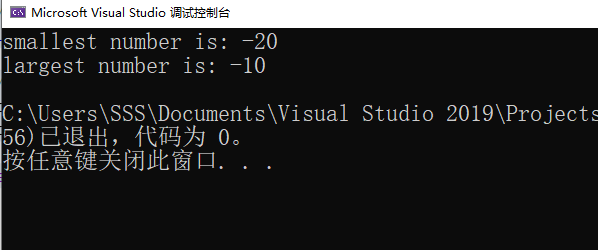展开全文• ## Matlab中max函数详解

万次阅读 2022-01-28 19:51:25
max函数用于获得单个向量中的最大值，示例如下： % Matlab A = 1:5; Am = max(A); 但是max函数还有另一种用法，那就是对两个向量逐元素比较大小，然后返回两个元素之间的较大值，示例如下： % Matlab A = 1:...matlab
• 对于MySQL中MAX函数如何使用这个问题，相信很多新手都不是很清楚，因此，为了能够帮助大家解决个难题，今日小杜就来为大家详细的介绍一下，希望大家看完之后有所收获，感兴趣的朋友不仿来看看。 首先，要了解MAX函数...mysql MAX函数
• Excel中MAX函数中的“*”起什么作用？连接作用，表示满足前面条件的对应D列数据。Excel中MAX是什么意思max是求最大值函数。它是excel函数中使用较高的常用函数，例如用来计算学生最高成绩、员工最高工资、以及最大...
• ## sql中的max函数

千次阅读 2022-04-16 08:36:37
想查询下订单数最多的客户号和...max聚合函数不能在select后面加其他细节， 若想要显示具体细节，可以加group by，但结果又不是我想要的。 所以用了嵌套子查询，这就可以啦。[face]emoji:023.png[/face] ...sqlserver
• MAX函数用于计算一组值中的最大值。MAX函数的语法如下：MAX(number1,number2,...)其中，参数number1、number2……是要从中找出最大值的1～255个数字参数。下面通过实例详细讲解该函数的使用方法与技巧。打开“MAX...
• 其实使用max函数就能很轻松的解决这个问题： 代码： str1 = "AAAaaa8888899sssss" print(max(str1, key=str1.count)) 结果：8 二、原理 max()函数用于获得给定的可迭代对象中的最大值。 key是max()函数的一...python
• max,min,sum函数的使用 QueryWrapper<MemberLevel> queryWrapper = new QueryWrapper<>(); queryWrapper.select("max(level_sort) as levelSort"); 相当于sql : select max(level_sort) as levelSort...java
• ## Max函数、Min函数

千次阅读 2021-04-11 15:58:47
Max函数：公式Max(范围)，Max（数值1,数值2） Min函数：公式Min(范围)，Min(数值1,数值2)
• python中的max() 方法返回给定参数的最大值，参数可以为序列。以下是 max() 方法的语法:max(x,y,z,....)参数x--数值表达式。y--数值表达式。z--数值表达式。例如print"max(80,100,1000):",max(80,100,1000)print"max...
• static int Max(int num1,int num2) 内部函数又称静态函数。在C++中使用内部函数，可以使函数只局限于所在文件。如果在不同的文件中有同名的内部函数，互不干扰。 C++外部函数 在C++中定义函数时，如果在编程语言
• max 数组的最大元素 1、M = max(A) 返回数组的最大元素。 如果 A 是向量，则 max(A) 返回 A 的最大值。 如果 A 为矩阵，则 max(A) 是包含每一列的最大值的行向量。 如果 A 是多维数组，则 max(A) 沿大小不等于 1 的...
• max和min函数的使用介绍： 对可迭代对象元素进行比较，找到最大/最小值 max(iterable, *[, default=obj, key=func]) 对传入的多个参数进行比较，找到最大/最小值 max(arg1, arg2, *args, *[, key=func]) 介绍对...
• 1. max() ...1) 第三个参数cmp可写可不写, max_element() 和 min_element() 默认是从小到大排列，max_element() 输出最后一个值, min_element() 输出第一个值，但是如果自定义的 cmp 函数写的是从.
• 这是2013年09月27日上午10点半写的。上简单的三个题目：1. 用C语言运行出：************************************Hello，World ！！************************...(二)自己定义一个maxin3的函数，写出判断三个整数大小...
• 我使用的是max函数，该函数使用lambda表达式返回Player类型的实例，其中players列表中的totalScore最大。def winner():w = max(players, key=lambda p: p.totalScore)函数正确返回具有最大totalScore的Player类型的...
• 本文，用max函数来找出数据中的最大值。工具/材料电脑python操作方法01从一串数字里面，找出最大值。max([5,6,7,8])02从字符串里面找出最大值。max('56789')这里返回的是一个str，不是int，而且是单个的数字形式。03...
• stl max函数 C ++ STL std :: max()函数 (C++ STL std::max() function) max() function is a library function of algorithm header, it is used to find the largest value from given two values, it accepts two...python java
•r语言 数据挖掘 机器学习 人工智能
• Linux内核函数中的max函数编写得非常优秀，让我忍不住要拿出来分析一番。传统定义max函数是#define max(X,Y) ((X) > (Y) ? (X) : (Y))。是不是觉得这样定义已经perfect了，那先看下面这个例子。 int x = 1, y = ...
• ## 详解 torch.max函数

千次阅读 2020-10-03 13:20:10
torch.max() 返回输入张量所有元素的最大值。 参数: input (Tensor) – 输入张量 例子： >>> a = torch.randn(1, 3) >>> a 0.4729 -0.2266 -0.2085 [torch.FloatTensor of size 1x3] >&...Pytorch
• ## torch.argmax函数学习

万次阅读 多人点赞 2020-12-07 20:21:57
argmax函数：torch.argmax(input, dim=None, keepdim=False)返回指定维度最大值的序号，dim给定的定义是：the demention to reduce.也就是把dim这个维度的，变成这个维度的最大值的index。 1）dim的不同值表示不同...
• ## matlab中max函数用法

千次阅读 2020-07-11 23:26:36
Matlab中max函数在矩阵中求函数大小主要有以下3种： (1).a=max(A); 返回一个数组各不同维中的最大元素。 如果A是一个向量，max(A)返回A中的最大元素。 如果A是一个矩阵，max(A)将A的每一列作为一个向量，返回一个行...matlab
• Pandas是基于NumPy 的一种工具，该工具是为了解决数据分析任务而创建的。Pandas 纳入了大量库和一些标准的数据模型，提供了高效地操作大型数据集所需的工具...原文地址：Python pandas.DataFrame.max函数方法的使用 ...
• c++minmax函数 C ++ STL std :: minmax()函数 (C++ STL std::minmax() function) minmax() function is a library function of algorithm header, it is used to find the smallest and largest values, it accepts ...python java
• #max(list) 用于返回列表元素中的最大值 当列表中种的元素都是字符串时，则比较第一个字符的ASCLL码 当列表中的元素都是数字的时候，则比较数字大小 当列表中的元素都是键值对的时候，则比较键的大小 当列表中的元素...python...